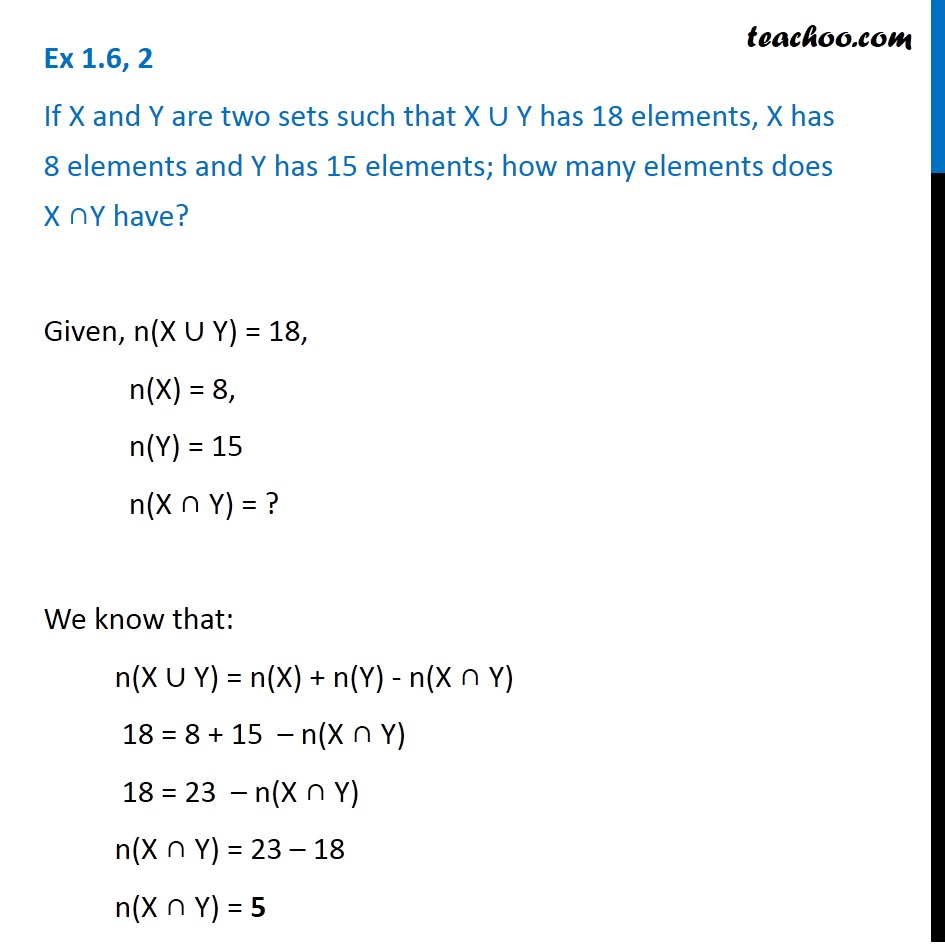1. Chapter 1 Class 11 Sets
2. Concept wise
3. Number of elements in set - 2 sets (Direct)

Transcript

Ex 1.6, 2 If X and Y are two sets such that X ∪ Y has 18 elements, X has 8 elements and Y has 15 elements; how many elements does X ∩Y have? Given, n(X ∪ Y) = 18, n(X) = 8, n(Y) = 15 n(X ∩ Y) = ? We know that: n(X ∪ Y) = n(X) + n(Y) - n(X ∩ Y) 18 = 8 + 15 – n(X ∩ Y) 18 = 23 – n(X ∩ Y) n(X ∩ Y) = 23 – 18 n(X ∩ Y) = 5

Number of elements in set - 2 sets (Direct)